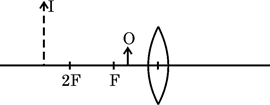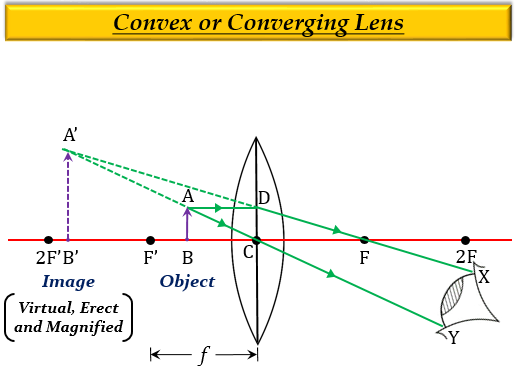Without actually drawing the ray diagram
">

# The diagram given below shows an object O and its image I.Without actually drawing the ray diagram

(i) Type of lens is Converging Lens (Convex lens) because the image is magnified (larger than the object) and on the same side as that of the object.

Explanation

When the object is placed between the optical centre $O$ and focus $F'$, then the image formed is virtual, erect, magnified, and on the same side as that of the object (on the left side).(ii) Magnifying Glass and Microscope are two optical instruments where such an image is obtained.

(iii) Three characteristics of the image formed if this lens is replaced by a concave mirror of focal length $f$ and an object is placed at a distance $\frac {f}{2}$ in front of the mirror is:

1. Virtual

2. Erect

3. Magnified

Updated on: 10-Oct-2022

414 Views